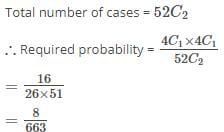# Test: Probability- 3

## 15 Questions MCQ Test IBPS PO Prelims- Study Material, Online Tests, Previous Year | Test: Probability- 3

Description
Attempt Test: Probability- 3 | 15 questions in 30 minutes | Mock test for UPSC preparation | Free important questions MCQ to study IBPS PO Prelims- Study Material, Online Tests, Previous Year for UPSC Exam | Download free PDF with solutions
QUESTION: 1

### In throwing a fair dice, what is the probability of getting the number ‘3’?

Solution:

Out of a total of 6 occurrences, 3 is one possibility = 1/ 6 .

QUESTION: 2

### Find the chance of throwing at least one ace in a simple throw with two dice

Solution:

The possible number of cases is 6×6, or 36.
An ace on one die may be associated with any of the 6 numbers on the other die, and the remaining 5 numbers on the first die may each be associated with the ace on the second die; thus the number of favourable cases is 11.
Therefore the required chance is 11/36

QUESTION: 3

### From a pack of 52 cards, two are drawn at random. Find the chance that one is a knave and the other a queen.

Solution:QUESTION: 4

Three coins are tossed. What is the probability of getting (i) 2 Tails and 1 Head

Solution:

(i) Event definition is: T and T and H or T and H and T or H and T and T = 3 x 1/8 = 3/8.

QUESTION: 5

Three coins are tossed. What is the probability of getting (i) neither 3 Heads nor 3 Tails?

Solution:

(i) Probability of 3 heads = 1/8 Also, Probability of 3 tails =1/8.
Required probability = 1- (1/8 + 1/8) = 6/8 = 3/4.

QUESTION: 6

What is the probability of obtaining at least one tail when a coin is tossed five times?

Solution:

‘At least one tail’ means that there can be one, two or three or four or five tails.
The only option that is not included is five heads.
The sum of all probabilities is always 1
P(at least one tail) = 1 - P(no tail)
P(no tail) = P(all heads) = P(H, H ,H, H ,H)
In any throw the probability of tail or head is ½
P(H, H ,H, H ,H) = 1/2 * 1/2 * 1/2 * 1/2 * 1/2       = (1/2)5
= 1/32
P( at least one tail ) : 1 - 1/32

QUESTION: 7

Two fair dice are thrown. Find the probability of getting

(i) sum of numbers divisible by 2 or 4.

Solution:

Positive outcomes are 2(1 way), 4(3 ways), 6(5 ways) 8(5 ways), 10 (3 ways), 12 (lway). Thus, 18/36= 1/2

QUESTION: 8

Three cards numbered 2, 4 and 8 are put into a box. If a card is drawn at random, what is the probability that the card drawn is

(i) a prime number?

Solution:

(i) Since 2 is the only prime number out of the three numbers, the answer would be 1/3

QUESTION: 9

Two fair dice are thrown. Find the probability of getting

(i) a prime number less than 8.

Solution:

Positive outcomes are 2(1 way), 3(2 ways), 5(4 ways), 7(6 ways). Total of 13 positive outcomes out of 36.
Thus, 13/36.

QUESTION: 10

A bag contains 3 green and 7 white balls. Two balls are drawn from the bag in succession without replacement. What is the probability that

(i) they are of different colour?

Solution:

White and Green or Green and White 7/10x39 + 3/10x7/9 42/90 = 7/15.

QUESTION: 11

In rolling two dices, find the probability (i) there is at least one ‘6’

Solution:

(i) With a six on the first dice, there are 6 possibilities of outcomes that can appear on the other dice (viz. 6 & 1, 6 & 2, 6 & 3, 6 & 4, 6 & 5 and 6 & 6). At the same time with 6 on the second dice there are 5 more possibilities for outcomes on the first dice: (1 & 6 , 2 & 6 , 3 & 6, 4 & 6, 5 & 6)
Also, the total outcomes are 36. Hence, the required probability is 11/36.

QUESTION: 12

Amit throws three dice in a special game of Ludo. If it is known that he needs 15 or higher in this throw to win then find the chance of his winning the game.

Solution:

Event definition is: 15 or 16 or 17 or 18. 15 can be got as: 5 and 5 and 5 (one way) Or 6 and 5 and 4 (Six ways) Or 6 and 6 and 3 (3 ways) Total 10 ways. 16 can be got as: 6 and 6 and 4 (3 ways) Or 6 and 5 and 5 (3 ways) Total 6 ways. 17 has 3 ways and 18 has 1 way of appearing. Thus, the required probability is:(10 + 6 + 3 + l)/216 = 20/216 = 5/54.

QUESTION: 13

In a horse race there were 18 horses numbered 1-18. The probability that horse 1 would win is 1/6, that 2 would win is 1/10 and that 3 would win is 1/8.
Assuming that a tie is impossible, find the chance that one of the three will win.

Solution:

1/6 + 1/10 + 1/8 = 47/120

QUESTION: 14

Two fair dice are thrown. What is the probability of (i) throwing a double?

Solution:

(i) There are six doubles (1, 1; 2 & 2; 3 & 3; 4 & 4; 5 & 5; 6 & 6) out of a total of 36 outcomes

6/36 = 1/6

QUESTION: 15

Two letters are randomly chosen from the word LIME. Find the probability that the letters are L and M.

Solution:

1/4C2 = 1/6.Use Code STAYHOME200 and get INR 200 additional OFF Use Coupon Code